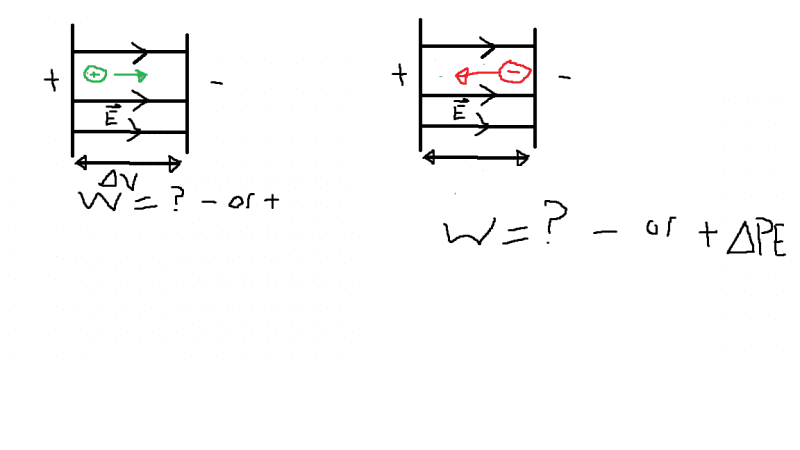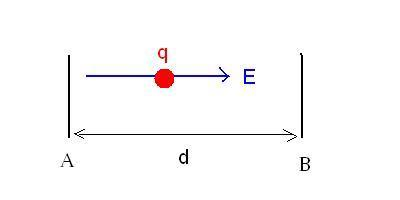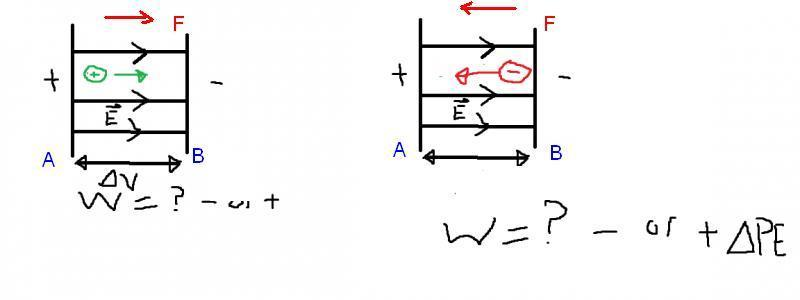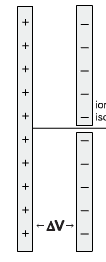# When does work=-electric potential and when does it = +

• sanhuy
In summary, when electric potential energy is applied between two parallel plates, work is done in order to move the positive charge from the left to the right side.

## Homework Statement

I have been trying to grasp the concept of work and electric potential energy inbetween two parallel plates with a uniform electric field and it is quite confusing. can someone explain when is work done = negative electric potential and when is it positive electric potential. i have been trying to figure this out for 3 days now please tell me straight forward.

## Homework EquationsThink like the only thing given is change in voltage and the charge.

## The Attempt at a Solution

I have looked throught textbook, online, this forums, and asked my professor. and no one has really helped me understand this concept. i know what potential energy ke and work is. i understand gravitational pe but not electrical. please someone explain.

sanhuy said:
Well, I write some more nonsense for you. I presume you can read ? As you misread what I wrote in my post.
That is what I have written in the other thread
Let we have capacitor plates A and B d distance apart. Let be the potential UA at A (on the left) and UB at B and UA>UB. The electric field is E=(UA-UB)/d, and points to the right, away from the positive plate. The force acting on charge q is F= qE, and the work done by the field when q moves from A to B is W=q(UA-UB). We define change of potential by Ufinal -Uinitial, and the change of potential energy is ΔEPE=q(Ufinal -Uinitial). Uinitial=UA, Ufinal=UB , so ΔEPE=q(UB-UA) which is opposite to the work done by the electric field. W = -ΔEPE.If you prefer using conservation of energy : The positive charge travels from A to B if UA>UB.
KE(A)+PE(A)=KE(B)+PE(B). PE(A)=qUA, PE(B)=qUB. -ΔKE=ΔPE=q(UB-UA). As UB<UA, UB-UA is negative. What is the sign of ΔKE?
Work-Energy theorem states that the change of KE equals to the work done. So is the work positive or negative?

thing is if we are only given Δv = +2500v how do you know if Ua > Ub or vice versa. I understand in potential energy of gravity you can set the initial height to zero can you set the initial voltage to 0 or final to zero. if so which one if change in voltage is +2500v? sorry for being an ***hole like i said in my first post, but if i can't understand something and you can its probably my fault for being so dumb.

sanhuy said:
thing is if we are only given Δv = +2500v how do you know if Ua > Ub or vice versa. I understand in potential energy of gravity you can set the initial height to zero can you set the initial voltage to 0 or final to zero. if so which one if change in voltage is +2500v? sorry for being an ***hole like i said in my first post, but if i can't understand something and you can its probably my fault for being so dumb.
If the particle goes from A to B the potential difference is UB-UA. You can set UA=0, but in case of positive Δv B is positive with respect to A. If A is positive with respect to B, the change of potential is negative.
Some people use"voltage" for negative potential difference. Look at the problem wording carefully, or ask if voltage is mentioned. How the original problem was worded?

Last edited:
so if the ΔV= +2500v like in a problem i am doing
I set point A to 0 and point B to +2500 since it is going from A to B it is single charged proton and i have to find final velocity at point B
so if i use
KEo+PEo = KEf+PEf
½×M×Vo2 + q(Ua) = ½×M×Vf2 +q(Ub)
0 + 0 = ½MVf2 + (1.6x10-2)(2500V)
-½MVf2 = (1.6x10-2[/SUPc])(2500V)
than Vf = √-2((1.6x10-2c)(2500V))

which is impossible.

my question tells use ΔV=2500v and "Motion of the positively-charged isotopes toward the right was initiated by a potential difference of 2500V on the two plates shown"sanhuy said:

my question tells use ΔV=2500v and "Motion of the positively-charged isotopes toward the right was initiated by a potential difference of 2500V on the two plates shown"[/SUP]
Potential difference means only the magnitude here. The clue is "Motion of the positively-charged isotopes toward the right was initiated by a potential difference of 2500V"The positive charge is repelled by the positive plate so which plate is positive with respect to the other if the positive particle is driven to the right?

Note the difference between potential difference and change of the potential. . Change of potential between an initial and final position is Ufinal-Uinitial. Is the final potential lower or higher than the initial one?

You know the water flows in the direction of lower potential. The same with positive charges. "By themselves" they move towards the lower potential.

so since the initial potential is high and protons flow to lower potential would the initial voltage be 2500v (Ua) or is that assumption invalid?

sanhuy said:
so since the initial potential is high and protons flow to lower potential would the initial voltage be 2500v (Ua) or is that assumption invalid?
Yes, the initial potential is 2500 V (with respect to the final one).

:):w:):):):):):):):):w:w:w:w:D:D:D:D:D

so if i did
½×M×Vo2 + q(Ua) = ½×M×Vf2 +q(Ub)
0+(1.6x10-19c)(2500v)=½×M×Vf2 + q(0v)
i would get 10/10?

wait if i did this with a negative charge going towards the positive terminal against the field. and ΔV= 2500v
i would get
This again Vf = √(2(-1.6x10-2c)(2500V))
the concept makes sense but math screws my concept up.

sanhuy said:
so if i did
½×M×Vo2 + q(Ua) = ½×M×Vf2 +q(Ub)
0+(1.6x10-19c)(2500v)=½×M×Vf2 + q(0v)
i would get 10/10?
10/10 what? What is the mass of the isotope?

ehild said:
10/10 what? What is the mass of the isotope?
0.666*10^-25kg but I am just asking if what i did was right.

and if i did it with a
negative charge going towards the positive terminal against the field. and ΔV= 2500v
i would get this again using conservation of energy eq KE + PE = KEf + PEf

Vf = √(2(-1.6x10-2c)(2500V)/M)
which won't work because of the negative.

edit: sorry forgot about dividing by mass

sanhuy said:
wait if i did this with a negative charge going towards the positive terminal against the field. and ΔV= 2500v
i would get
This again Vf = √(2(-1.6x10-2c)(2500V))
the concept makes sense but math screws my concept up.
If a negative charge goes toward the positive terminal then its potential energy decreases, as PE=qU and q is negative.
Initial position: UB=0, PEinitial=0, KE initial = 0.
Final position: UA=2500 V, PEfinal =[ -1.6x10-19 C](2500 V), KEfinal+Pfinal=0.--> -1.6x10-19 *2500 +1/2 Mv2=0.
Is there any problem?

sanhuy said:
0.666*10^-25kg but I am just asking if what i did was right.

and if i did it with a
negative charge going towards the positive terminal against the field. and ΔV= 2500v
i would get this again using conservation of energy eq KE + PE = KEf + PEf

Vf = √(2(-1.6x10-2c)(2500V)/M)
which won't work because of the negative.

edit: sorry forgot about dividing by mass
What you did with the positive charge was good. What you did with the negative charge, it was wrong.

I love you.

sanhuy said:
so since the initial potential is high and protons flow to lower potential would the initial voltage be 2500v (Ua) or is that assumption invalid?
Wait Wait Wait IF the initial voltage is 2500v and final is zero wouldn't that make ΔV = -2500v
instead of ΔV=+2500v like the question gave us.
(when proton goes from A to B)

sanhuy said:
Wait Wait Wait IF the initial voltage is 2500v and final is zero wouldn't that make ΔV = -2500v
instead of ΔV=+2500v like the question gave us.
(when proton goes from A to B)
Potential difference between points A and B is not the same as the change of potential along the route of a particle. The first refers too the set-up. The second refers to the moving particle.

The potential difference of point A with respect to point B is 2500 V. The problem said that there is 2500 V potential difference between the plates, but it did no say in what sense. You had to find it out, from he sentence, "Motion of the positively-charged isotopes toward the right was initiated by a potential difference of 2500V". Potential difference meant the magnitude of the difference between the potentials here.
If the positive isotope moved from the positive plate to the negative one, the potential changed from 2500 V to 0 V along its route, it traveled across -2500 potential difference. U final -U initial = -2500 V.